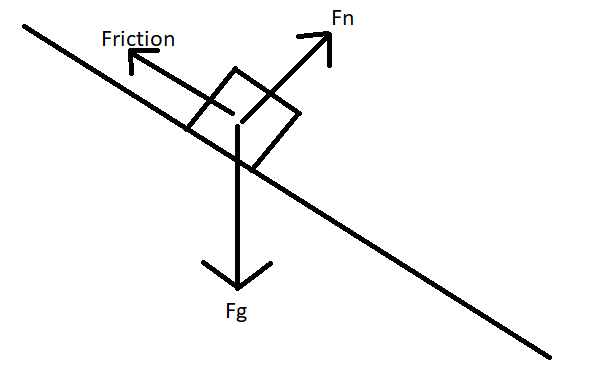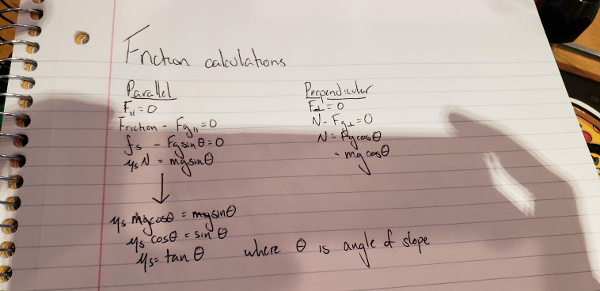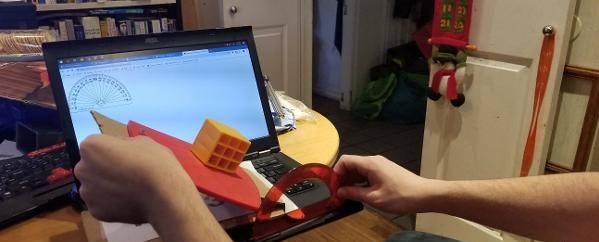# Friction Coefficient and Energy

Tags: think and design
Personhours: 1
Friction Coefficient and Energy By Ethan

### Task: Measure the coefficient of friction of our oven mitt intake

We want to measure various constants of materials on our robot. Earlier this season, we found that a nylon-mitt collision on our intake sapped the rotational energy of our intake. But, that was just a build error, easily fixable. But now, we plan to measure the energy lost from particle-mitt collisions, and the first part of this is to find the coefficient of friction of the silicone mitts.To measure the coefficient of friction, we first had to simplify an equation to determine what values to measure.From these calculations, we determined that the only factor to measure to determine the coefficient of friction between blocks and the mitts is the angle of incline. Therefore, we created a simple device to measure the angle at which slippage begins to occur.The angle was about 27 degrees, so the coefficient of friction is equal to arctan(27)=0.44. This is a pretty good coefficient of friction, meaning that the intake is very efficient in bringing the particles in, but it also means that the intake loses more energy on collision.

### Next Steps

We need to measure further constants such as stretch and wear of nylon. To do so, we're printing a simple testing nylon block.

Date | December 1, 2018